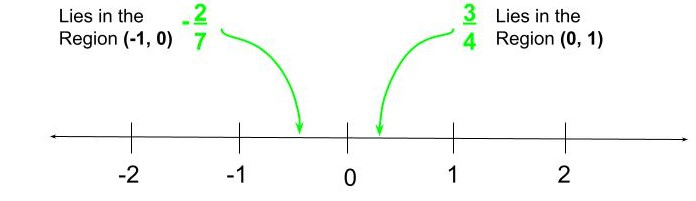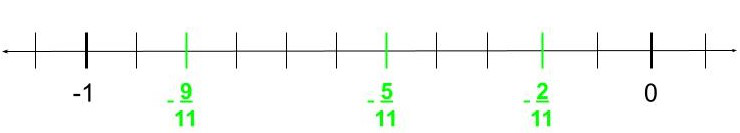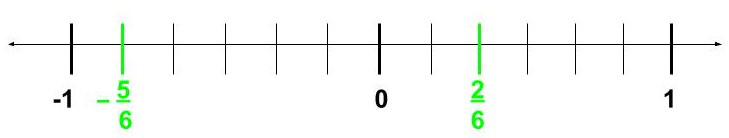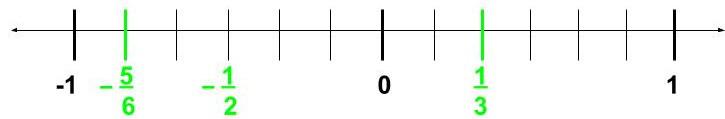Represent −2/11, −5/11, and −9/ 11 on the number line

• Last Updated : 03 Sep, 2021

A number is a value that is used as a standard to quantify or rather to say specify the quantity of objects and also perform mathematical calculations.

A Number System is defined as a system of writing to express numbers that belong to the same group or share some common features or properties. It is the mathematical notation for representing numbers using a particular set of digits expressed in a logical and consistent manner. It provides a unique method to represent every number and represents the arithmetic and algebraic structure of the figures.

It also allows us to perform arithmetic operations like addition, subtraction, multiplication, and division.

The value of any digit in a number can be determined by:-

• The digit (Ex: 0, 1, 2)
• Its position in the number (i.e. whether the digit is in the tenth place or hundreds place or thousands place, etc. Also whether the digit lies in the left or the right side of the decimal point)
• The base of the number (Ex: Base can be 2 for a binary number and 10 for a decimal number)

There are 4 types of Number Systems. Namely:

• Binary number system (Base – 2)
• Octal number system (Base – 8)
• Decimal number system (Base – 10)
• Hexadecimal number system (Base – 16)

Here we will be focusing on the Decimal Number System with base 10.

The base 10 means that we can use digits from 0 to 9 to represent a number. We can use the combination of the digits 0, 1, 2, 3, 4, 5, 6, 7, 8, 9 to form a decimal number with base 10. For example, 3541 is a number that can be represented on a Number Line of a Decimal Number System.

If we break down 3541, it can be easily represented in the form:

3 × 103 + 5 × 102 + 4 × 101 + 1 × 100 = 3541

What is a Number Line?

A Number Line is nothing but a way of representing numbers on a straight line where all numbers have to belong to or be a part of a particular Number System. Here numbers have to be placed in fixed regular intervals and the spacing between a pair of consecutive numbers and the difference of values between the two numbers must be maintained all through a particular Number Line.

However, different Number Lines can have a different set of rules, unique and exclusive for spacing and difference of values between any two consecutive numbers where all numbers belong to some particular Number System.

The numbers can be whole numbers, integers, rational numbers provided the spacing and difference of values are maintained throughout the Number Line.

If we arbitrarily put a point on a Number Line, then numbers, or rather to say values of the numbers increases as we move along the right of the specified point, and values of the numbers decrease as we move left from the point along the Number Line.

What are Rational Numbers?

A rational number is a real number belonging to the Decimal Number System that can be represented on a Number Line and can be expressed in the form P/Q. Here P and Q are integers where P can have any integral values but Q cannot be 0. If Q = 0, then P/0 becomes undefined. However, P can be 0 as 0/Q is still a valid real number that equals 0.

Any real number can be expressed in the form of P/Q. For example, the integer 8 can be represented in the form 8/1 and the value still remains the same. Also, P can be greater than or less than or equal to Q. Rational numbers can be positive, 0, negative.

Represent -2/11, -5/11, and -9/11 on the Number Line

To represent rational numbers on a number line, we first need to check the values of the numerator and denominator of the rational number.

For example, if we take a number 3/4, then the numerator (N) is 3 and the denominator (D) is 4.

If  N < D, and they are co-prime, then

The rational number lies in the region between 0 and 1 if the number is positive and in case it is negative, then it lies between 0 and -1 on the number line.

So, if we consider two rational numbers 3/4 and -2/7 then,The denominator (D), depicts the number of parts the region will get divided into.

The numerator (N), depicts the nth number part from 0 and move right on the number line if the number is positive and move left if the number is negative.

The numbers -2/11, -5/11, -9/11, all of them have common denominator i.e. D = 11. Since all the numbers are negative, therefore all of them lie in the region (-1, 0) on the Number Line.Representation of -2/11, -5/11, -9/11 on the Number Line

As D = 11, so we divide the portion of the number line in the region (-1, 0), into D equal (here 11) parts.

To place the number -2/11 we look at the numerator value. Since the numerator N = 2, we move two equal parts away from 0 and in the left direction. Similarly to place -5/11, we move 5 equal parts away from 0 and so on.

Similar Questions

Question 1: Represent -5/6 and 2/6 on the Number Line

Solution:

Here, both -5/6 and 2/6 have a common denominator, i.e. D = 6.

Now -5/6 is negative, so it lies on the left side of 0, i.e. in the region (-1, 0). Whereas 2/6 is positive, so it lies on the right side of 0, i.e. in the region (0, 1). So, we divide the region (-1, 0) into six equal parts and repeat the same for the region (0, 1).Representation of -5/6 and 2/6 on the Number Line

Question 2: Represent -5/6, -1/2, and 1/3 on the Number Line

Solution:

Here, we can see the rational numbers do NOT share a common denominator.

In such cases, we need to find the LCM of all Denominators. For example, here the L.C.M of 6, 3 and 2 is 6.

Now, we have to represent or express the rational numbers in such a way that all of them share a common Denominator.

-(5 * 1)/(6 * 1) = -5/6

-(1 * 3)/(2 * 3) = -3/6

(1 * 2)/(3 * 2) = 2/6

So, we get the rational numbers -5/6, -3/6 and 2/6. All of them share a common Denominator, i.e. D = 6.

So, now we can easily place the rational numbers on the Number Line and after placing them replace with the original value.Representation of -5/6, -1/2 and 1/3 on the Number Line

My Personal Notes arrow_drop_up